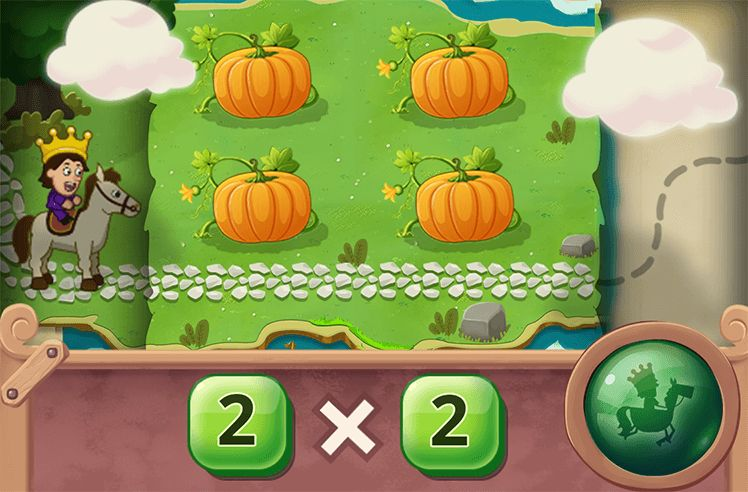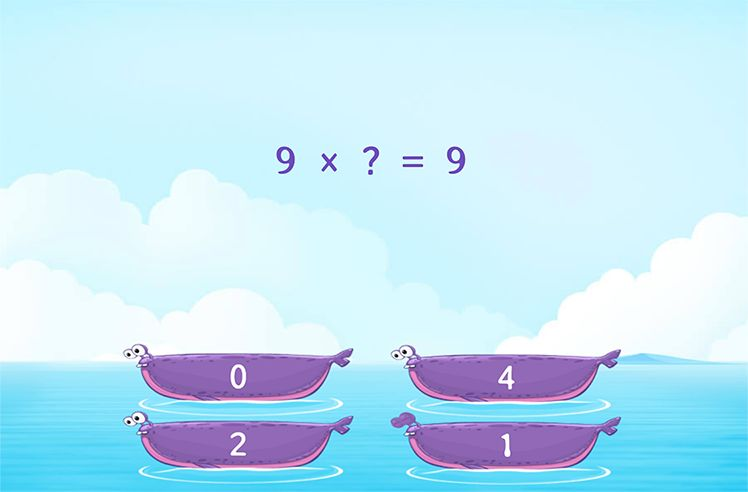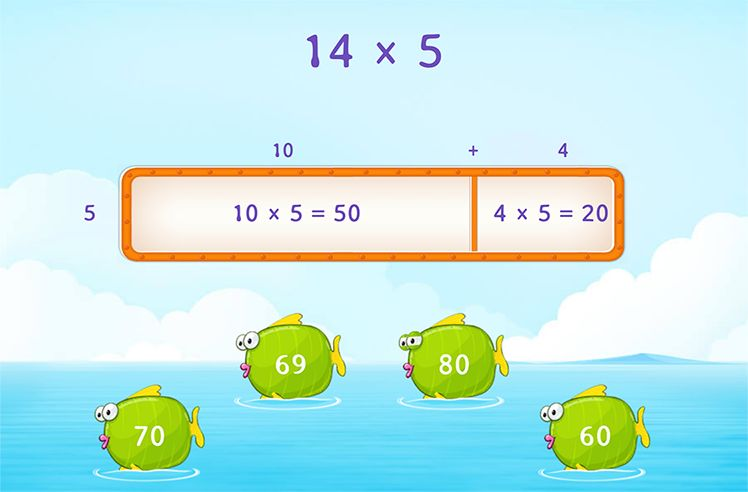# Multiplicative Identity Property of 1 – Definition with Examples

» Multiplicative Identity Property of 1 – Definition with Examples

## What is the Multiplicative Identity Property of 1?

According to the multiplicative identity property of 1, any number multiplied by 1, gives the same result as the number itself. It is also called the Identity property of multiplication, because the identity of the number remains the same.

Here are some examples of the identity property of multiplication.

##Let’s sing!

Multiplying numbers by 1 is fun like a gameBecause the product will just be the same!

##Let’s do it!

Play a game with your child. Ask him/her to be as prompt as they can be  while replying, when you ask them to multiply big and small numbers with 1.

##Related Math VocabularyIntroduction to Multiplication

Play NowMultiplication Properties

Play Now